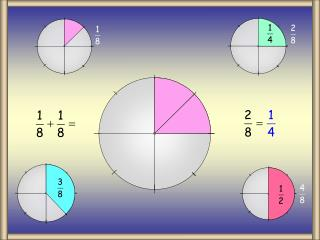DownloadDownload Presentation1/8 + 1/8

# 1/8 + 1/8

Download Presentation## 1/8 + 1/8

- - - - - - - - - - - - - - - - - - - - - - - - - - - E N D - - - - - - - - - - - - - - - - - - - - - - - - - - -
##### Presentation Transcript

1. 1/8 + 1/8

2. 1/8 + 1/4

3. 1/4 + 1/4

4. 1/2 + 1/8

5. 1/2 + 1/4

6. 3/8 + 1/4

7. 3/8 + 3/8

8. 1/2 + 3/8

9. 8 Sector Blank

10. Worksheet

11. 12 Sector Circle

12. 1/2 + 1/12

13. 1/2 + 1/6

14. 1/2 + 1/4

15. 1/2 + 1/3

16. 1/3 + 1/12

17. 1/3 + 1/6

18. 1/3 + 1/4

19. 1/4 + 1/6

20. 1/4 + 1/12

21. 1/6 + 1/12

22. 12 Sector Blank 1/6 + 1/12

23. Worksheet

24. Num/Den Numerator REMEMBER: Denominator Addition/Subtraction of Fractions Adding/subtracting fractions with the same denominator is easy. You simply add/subtract the numerators.

25. Same Den Adding and subtracting fractions with the same denominator is easy. You simply add or subtract the numerators.

26. LCM Find the lowest common multiple of the following numbers: Multiples of 3 and 8 3 and 4 5 and 20 3 and 8 3 8 Multiples of 3 and 4 Multiples of 5 and 20 6 16 5 3 20 4 9 24 6 10 40 8 12 32 9 15 60 12 15 40 20 12 16 80 18 48 15 25 100 20 21 56 12 is the LCM 24 64 20 is the LCM 24 is the LCM Adding/subtracting fractions with different denominators is more complicated. You need to find the Lowest Common Multiple (LCM) of the denominators first. Remember: The Lowest Common Multiple of 2 or more numbers is the smallest number that the given numbers will divide into without remainder.

27. + Equivalent Multiples of 3 and 4 Equivalent 3 4 6 8 9 12 12 16 15 20 12 is the LCM + Diagram 1 + =

28. + Diagram 2 + Equivalent Multiples of 5 and 8 Equivalent 5 8 10 16 15 32 20 40 25 48 30 56 35 64 40 72 40 is the LCM + =

29. + Diagram 3 + Equivalent Multiples of 4 and 6 Equivalent 4 6 8 12 12 18 16 24 20 30 12 is the LCM + =

30. Examples + 1 Example questions 15 + 8 1 24 + 5 8 2 20 + 9 1 3 12

31. Examples + 2 Example questions + 6 5 4 8 + 33 14 5 36 + 27 40 6 72

32. - Diagram 1 - Equivalent Multiples of 3 and 4 Equivalent 3 4 6 8 9 12 12 16 15 20 12 is the LCM - =

33. - Equivalent Multiples of 3 and 4 Equivalent 3 4 6 8 9 12 12 16 15 20 12 is the LCM - =

34. - Diagram 2 - Equivalent Multiples of 5 and 8 Equivalent 5 8 10 16 15 32 20 40 25 48 30 56 35 64 40 72 40 is the LCM - =

35. - Equivalent Multiples of 5 and 8 Equivalent 5 8 10 16 15 32 20 40 25 48 30 56 35 64 40 72 40 is the LCM - =

36. -Diagram 3 - Equivalent Multiples of 4 and 6 Equivalent 4 6 8 12 12 18 16 24 20 30 12 is the LCM - =

37. - Equivalent Multiples of 4 and 6 Equivalent 4 6 8 12 12 18 16 24 20 30 12 is the LCM - =

38. Examples - 1 Example questions 15 - 8 1 24 - 5 8 2 20 - 1 9 3 12

39. Examples - 2 Example questions - 6 5 4 8 - 14 33 5 36 - 27 40 6 72